﻿ Foreign Direct Investment and Total Factor Productivity Growth Nexus: A Realistic Testimony from VECM ApproachPublications are Open
Access in this journal
Article Versions
Export Article
• Normal Style
• MLA Style
• APA Style
• Chicago Style
Research Article
Open Access Peer-reviewed

### Foreign Direct Investment and Total Factor Productivity Growth Nexus: A Realistic Testimony from VECM Approach

Justicia Nanah Allie , Isaac Adjei Mensah
Journal of Finance and Economics. 2019, 7(4), 118-126. DOI: 10.12691/jfe-7-4-2
Received October 02, 2019; Revised November 15, 2019; Accepted November 28, 2019

### Abstract

The main focus of current paper is to investigate the effect of foreign direct investment (FDI) on growth of total factor productivity (TFP) of Sierra Leone using financial development (FD) and trade openness (TR) as intermittent variables for the period 1990-2016. Further, this paper intends to identify the direction of causal affiliations amid employed series. Base on the application of ADF and PP-Fisher unit root tests as well as JJ co-integration tests respectively, all variables used are stationary at the first difference and also co-integrated. Through the estimation a proposed TFP model using the VECM framework, FDI is evidenced to have a significant positive effect on TFP when all other factors are held constant. Findings from the Granger causality test further revealed unidirectional liaison from FDI to TFP in the long-run whilst bidirectional causality is evidenced in the short-run. We therefore conclude that in order to increase the positive externalities and spillover benefits of FDI at the corporate level, Sierra Leone could provide assistance to foreign investment companies in management and worker training.

### 1. Introduction

Nearly 30 years, foreign direct investment has been a highlight of the world economy. As an important factor of globalization, foreign direct investment (FDI) has attracted more and more attention in recent years and aroused the interest of policy makers and academic researchers. This interest in foreign direct investment is generally attributed to the employment-generating capacity of information technology its impact on productivity growth and its dynamic link with competitiveness. Foreign direct investment improves the general welfare of the people by providing jobs and accelerating economic growth. It also contributes to the adoption of new methods of production and increases productivity by bringing more competition to the economy. Foreign direct investment is seen as a tool for transferring skills, technology and knowledge reserves between countries. Therefore, FDI is regarded as an important channel for international knowledge transfer 1.

In the past 30 years, although the developed countries and developing countries has quite a lot of evidence to prove the connection between the foreign direct investment and economic growth, but the TFP growth and empirical survey of the relationship between foreign direct investment are few and far between. Even if one accepts the positive correlation between the variables in question, there is still a causal relationship between them. In Sierra Leone, the causal relationship between these two variables remains an important issue, so it is even more important to explore the relationship between FDI and TFP growth. It is generally believed that FDI is positively correlated with the growth of TFP. However, much empirical and theoretical research has been done on the link between TFP growth and FDI in Sierra Leone. Therefore, the main purpose of this study is to use trade liberalization and financial development as control variables during the period 1990-2016 to estimate the contribution of FDI to TFP and the direction of its causal relationship. The reason why the authors of this study chose Sierra Leone is that, to our knowledge, not much has been done to explore the causal relationship between foreign direct investment and economic growth. Therefore, this study attempts to fill this gap. The remainder of the paper is organized as follows; Section 2 briefly looks at the existing literature linking FDI to TFP growth; Section 3 outlines the various methods used in the study; Section 4 presents the discussion of empirical results while Section 5 finally concludes the study with policy implications.

### 2. Review of Empirical Literature

Since this paper focuses on the relationship between TFP growth and FDI in developing Sierra Leone, the author makes a brief empirical review of some previous literature in this field, rather than discussing the literature on FDI determinants. While this link can be studied at the micro level or the spillover effects of FDI within a sector we will focus on the overall level. So far, few studies have examined the impact of FDI on TFP at the macro level, and the results are mainly positive; however, some authors suggest that variables may be negatively correlated. We will discuss both options and begin to investigate the situation in Sierra Leone.

For a long time, TFP has been considered as an exogenous variable in the regular output model. However, as noted by 2, this is not observed in an open market economy. In his study of the endogenous nature of TFP, he found that integration can promote growth because integration into world markets means openness and the possibility of investment from abroad and receiving investment. A corollary of this finding is that FDI, as a channel for capital to flow between economies, also leads to increased productivity growth, similar to the efficiency gains achieved by trade. Critically, 3 looked at the impact of FDI on TFP and economic growth for Turkey during the period 1980-2010. His empirical results are only based on the regression of FDI to TFP and GDP, indicating that FDI has a positive impact on both through technology spillover and capital accumulation. 4 obtained positive results from the OECD country expert group and concluded that FDI had a positive impact on TFP in the host country. He attributed this to foreign direct investment as a channel for the international transfer of technology. In addition, 5 showed that FDI had a positive impact on TFP growth in a large sample of countries from 1970 to 2010.

The positive link between TFP and FDI varies in nature, particularly for developing countries and economies in transition. For example, 6, studied the inter-regional analysis of FDI's contribution to productivity growth in China from 1994 to 2010, and found a two-way causal relationship between FDI and TFP. The results show that China's economic growth owes much to the rapid expansion of physical investment in fixed assets from FDI, rather than technology transfer. This is the result of inefficiency and a lack of capacity to absorb technology. Nevertheless, foreign direct investment in labor-intensive sectors did have a positive impact on labor productivity. Even if a positive correlation is shown between variables, developed countries seem to experience the effects of FDI in a different way than developing countries. The research of 7 on American factories (1990-2015) shows that FDI effect is more obvious in technology-oriented industries due to better communication with international companies. More than 10 per cent of productivity growth is due to spillovers from foreign direct investment. The more organized and advanced an economy, the more concrete the impact of foreign direct investment seems to be. Similarly, the authors' view of positive relationships may vary from country to country. 8 estimated the impact of FDI on capital accumulation, output and TFP growth, and concluded that the impact of FDI was country-specific, which was due to factors that could not be observed in time series analysis. The impact of FDI depends on whether the recipient country is a leader or a laggard since the impact of technology transfer is lower in developing countries. These observations are based on time series panel data from a sample of OECD and non-OECD countries for the period 1970-1990.

FDI has no direct positive impact on TFP, which is usually interpreted as the low efficiency of economic absorption, making the country unable to benefit from any growth of human capital and technology 9. In addition, the degree of economic freedom, economic openness and the establishment of an effective financial environment also play an important role. For example, a study by 10, shows that there is a negative correlation between FDI and TFP. For several developing Arab countries (Egypt, Jordan, Morocco, Oman, Saudi Arabia and Tunisia), they investigated whether FDI affected TFP through technology spillovers and found that FDI actually had a "very noteworthy and negative upshot" on most of the countries included in the study. However, the authors make it clear that the reasons for this may be the inefficiency of government policies and institutions, the inefficiency of investment, and the lack of added value and availability of technological innovations. In view of the inconsistency of the literature, a model of the influence of FDI on TFP was proposed. However, other variables that might affect TFP are not excluded. To date, most studies have included additional variables expected to have a positive effect on TFP; nevertheless, we have strong-willed to include economic factors that may have a negative impact on TFP.

To sum up, the endogenous growth prose presupposes that the knowledge and technology overflow of FDI on the host country has more long-term growth potential, most findings support ability enhancement effect of FDI growth of human capital point of view. In view of this, the stock of human capital has increased total factor productivity growth by promoting domestic innovation and accelerating the pace of technological catch-up in leading countries. Given the limited technological capacity of most developing countries, FDI can contribute to technological change through the transfer of knowledge and technology, which in turn can be strengthened by the human capital stock of the recipient countries. Finally, it can be concluded that a friendly foreign direct investment regime remains the necessary and basis for attracting investors, particularly in most developing countries, such as Sierra Leone. This is because contemporary investors are looking for viable locations and are prepared to implement appropriate competition laws, transparent rules, and sustainable incentive frameworks and productive resource pools for private and public enterprises to drive their economic growth.

### 3. Methodology

3.1. Data Source and Description

The study uses a single cross-country time series data covering the period of 1990 to 2016 for the variables foreign direct investment (FDI), total factor productivity (TFP) growth, financial development and trade openness (TOP) together with human capital stock, labor and physical capital stock. The data with respect to the aforementioned variables are obtained from World Bank Development Indicator 5. The data per each variable is transformed into natural logarithm in order to the parameters estimates elasticities of the response variable and as well reduce the issues of heteroskedasticity. Despite the fact that several measures of FDI have been used in literature, this recent paper uses the stock of FDI rather than FDI on the economy as a whole. Moreover, FDI stock is preferable over FDI inflows in the sense that, in economic theory it is more appropriate to concentrate on the accumulated stock of FDI rather than focusing on the inflows of FDI since FDI stock ultimately determines the marginal productivity of factors. Physical capital stock (K) on the other hand is constructed by using the perpetual inventory methods of 11 where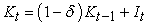with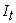defined as investment or gross fixed capital formation and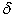represents the depreciation rate. The initial physical capital stock (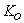) therefore is computed using the relation: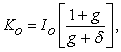where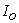is the initial investment, and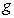is the real GDP growth rate in the long-run. Per this current work, real GDP which is also obtained from WDI is measured in constant 2010 US\$. Human capital in this case is defined as augmented labor which is estimated as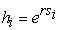where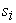is the average year of schooling of the active population and r is the date of return to schooling which is set to 10%. Labor (L) which is measured as the volume of the total labor force whereas financial development (FD) and trade openness (TOP) are defined as total credit to private sector as a ratio of GDP and exports plus imports as share of GDP respectively. The overall period together with the selection of variables for the current study are dictated by data availability.

3.2. Theoretical Model Specification

With the main purpose of investigating the impact of foreign direct investment (FDI) on the growth of total factor productivity (TFP) in Sierra Leon, the following Hicks Neutral Cobb-Douglas production function is employed where growth output Y(t) is expressed as function of physical capital stock (K), human capital (H) and labor (L) illustrated in Equation (1) as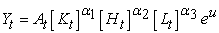(1)

where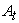is the level of TFP whilst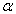and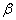demote the elasticities of domestic physical capital and human capital.

From the model in Equation (1),which measures the level TFP, is allowed to be endogenously determined by financial development, trade openness and foreign direct investment within an extended Cobb-Douglas production function. The model therefore can be written as;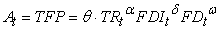(2)

where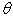is time-invariant constant, TR is an indicator for trade openness, FDI is foreign direct investment and FD is financial development. Taking natural logarithm of the Equation (2) gives the following as Equation (3);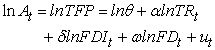(3)

By substituting Equation (2) into Equation (1) we obtain the production function in an extension form as;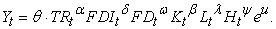(4)

Taking natural logarithm of Equation (4), the linearized Cobb-Douglas function is given by: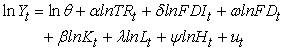(5)

where,,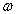,,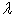, and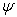captures the effect of trade openness, foreign direct investment, financial development, physical capital, human capital and labor on growth output.

Equation (3) and Equation (4) can therefore be used to the estimate the effect of FDI on TFP and growth output respectively.

In order to estimates TFP using the growth accounting framework as according to 3, The variableas depicted in Equation (1) captures the TFP effect on growth output and it is assumed that the effect of FDI, TR and FD on growth operates through variable A and the effect of FDI, TR and FD on A also depends on the human capital (H). As the current study centers on the growth of TFP level, it is of importance to formulate Equation (6) in terms of output per capita. Thus TFP in this perspective is estimated as;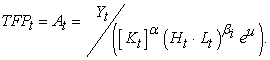(6)

Alternatively, the level of TFP can also be calculated by additionally taking natural logarithm of both sides of Equation (6) to obtain: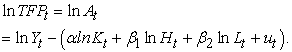(7)

Using this model, the level of TFP is computes or estimated by subtracting the contributions of factors of production from the growth output.

3.3. Econometric Approach

This current study adopts a quantitative research design with descriptive and econometric methodologies. A multivariate time series model is developed. The study examined the stationarity properties of the variables within the times series data using the Augmented Dickey-Fuller (ADF) and Philips and Peron (PP) unit root tests, as a starting point for the analysis, so as to avoid spurious regression results. After confirming the stationarity properties of the analyzed variables within the data, a 12 cointegration test was used in the next step to examine the existence of long-run relationship amid variables within the data. In estimating the structural long-run relationship amid the analyzed variables, the study further employed the vector error correction model (VECM) test and finally tested for the direction of causalities amid these variables by making use of the Granger causality test based on error correction framework. Brief details of employed econometric techniques are as follows;

3.3.1. Unit Root Test

The first step before examining the cointegration is to test the stationarity of the time series variables. A linear combination of two non-stationary series can be stationary and if such stationarity exists then the series are said to be cointegrated. This thus requires that the series have the same order of integration. Hence, the Augmented Dickey and Fuller (ADF) and the Phillip and Perron (PP) unit root tests developed by 15 and 16 are performed to determine whether the data are stationary at difference or trend stationary and to also determine the number of units at their levels. Both the ADF and PP unit root test are used to test for the null hypothesis of unit root (non-stationarity) against no unit root (stationarity) as alternative hypothesis.

3.3.2. Cointegration test

In the case where analyzed variables are identified to be non-stationary at their respective levels and are integrated in the same of order that is order one or more, a cointegration test is applied further to examine the existence of long-run relationship. As such this current study as mentioned earlier utilized the J-J cointegration test developed by 17, beginning with an unrestricted vector auto-regressive (VAR) regression model which contains a vector of variables at time t are related to vector of past variables. In accordance to the Granger representation theorem, the vector of variables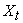consist of a VAR error correction representation in the following specification;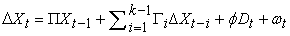(8)

where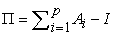and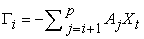is a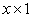dimension vector corresponding to the number of variables used in the study in which all the variables are integrated at order one (I(1)),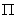,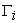and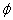are estimated parameter matrices,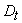represents a vector with deterministic elements (constant and trend) and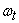From Equation (8), there cannot be any relationship amid a variable of I(1) and I(0). Hence, if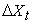is integrated at order zero (I(0)) then it implies thatwill be zero if only a linear combination of the variables inare permissible from the specified model of the study. Also if the rank ofis equal to(rank () =), thenis invertible and all the variables in the levels are stationary implying there is no cointegration existing. Further if rank ofis equal to 0 (rank () =0) indicating all elements in the adjustment matrix have value zero, then none of the linear combinations is stationary. Thus an unrestricted VAR model can be estimated in order to identify the short-run dynamics only. Based on the Granger representation theorem, of 0< rank (=r) <, there exits r cointegration vectors or in other words r stationary linear combinations. The existence of cointegration in the model takes out possibilities of spurious relationships between the non-stationary variables. For results consistency, two estimation methods of JJ cointegration test are used which includes the trace statistics and the maximum Eigen value statistic. Both statistics are given respectively by the relations;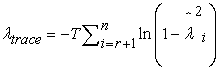(9)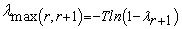(10)

where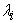is the estimated ordered Eigen value computed from the estimated matrix and T is the number of observations useable after the adjustment of lags.

The statistic based on the trace tests the null conjecture that the number of distinct cointegration vector (r) is not more than or the same as r versus the general alternative hypothesis. On the other hand, the maximum Eigen value tests the null hypothesis that the number of cointegration vector is equal to r versus the alternative of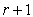co-integrating vector.

3.3.3. Vector Error Correction Model (VECM) Test

Once the JJ co-integration test rejects the null hypothesis that there exist a structural long-run relationship in the model or in other words as all series in the model are non-stationary and cointegrated, the vector error correction model (VECM) test could further be conducted in order to identify (estimate) the direction of the long-run relationships. Equation (11) therefore depicts the VECM regression used for the model proposed for the study using TFP growth as the response variable: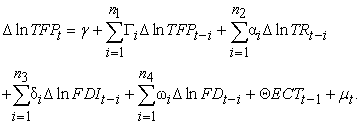(11)

where; TFP, TR, FDI, FD and ECT represent, total factor productivity, trade openness, foreign direct investment, financial development and error correction term.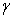on the other hand is the intercept, n is the number of lags,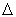is the difference estimator, ln is the natural logarithm.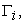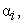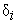and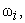are long-run coefficients which have the causality information for TFP, TR, FDI, and FD respectively and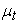is the residual term.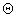also measures the estimated effect of the model’s speed of adjustment from the short run to long-run. This means that, the magnitude and the statistical significance of a one period lag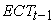coefficient measures how fast the disequilibrium in TFP is corrected in order to return to equilibrium.

From Equation (11) above, n is the number of lags, Schwarz information criterion (SIC) set lag number one to the optimal lag. The VECM equation becomes as follows in Equation (12);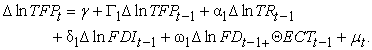(12)

From Equation (12), the response variable TFP is regressed on its past value and on past values of other variables.

3.3.3. Granger Causality in the Framework of Error Correction Model (ECM)

After the co-integration relationship is affirmed and estimated from the JJ cointegration test and VECM test in the long-run respectively, the study further used the Granger causality test in an error correction modeling framework. The existence of cointegration in the relationship between variables implies long-run causality at least in one direction which under certain restrictions can be tested by using the Wald test 13. In order to be able to identify both the short-run and long-run causal relationships among the analyzed variables, Equation (11) can be reformulated in the case of multivariate models of total factor productivity, trade openness, foreign direct investment and financial development as;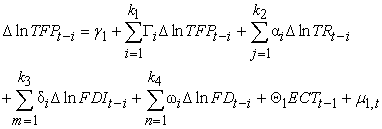(13)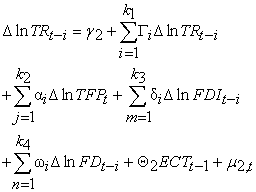(14)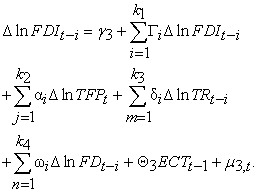(15)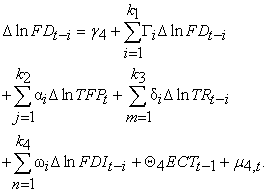(16)

### 4. Results and Discussions

4.1. Summary of Descriptive Statistics, Correlation Matrix and Multi-colinearity

A brief summary of the descriptive statistics is presented in Table 1. With respect to our findings, the most important series refers to the actual deviation from the mean value of the variables proposed in the study. To be more specific, the value of the standard deviation for the response variable (TFP growth) is equal to 7.693 with s standard deviation of 1.914. Furthermore, the same statistics for trade openness (TR), foreign direct investment (FDI) and financial development (FD) are respectively obtained as 5.848 (0.211), 6.346 (1.592) and 6.190 (1.552), where those in parenthesis represents the corresponding standard deviations. Further, Table 1 gives the value on Skewness, kurtosis, as well as JB tests which helps to verify whether the series with the employed data follows the normal distribution. It can therefore be deduced that the response variable TFP together with the independent variables are negatively skewed with the exception of TR which is flattened to the right (positively skewed) compared to the normal curve. Also the kurtosis value of TFP being the dependent variable in the study is evidenced to be greater than the normal value (3). This gives the indication that, the shape of this distribution is leptokurtic. The explanatory variables which includes FDI and FD are found to have a mesokurtic shape in the sense that they respectively have their kurtosis values which are approximately 3 whereas TR is the only variable evidenced to be platykurtic in terms of shape since its value of kurtosis is approximately less than 3. Generally, the normal value of the Skewness is “zero” and that of kurtosis is “three” when the observed series is normally distributed. The result per the kurtosis and the Skewness for the various variables used in the study is in line with the Jarque-Bera test statistics in which all its values are not approximately zero or exactly zero. The JB test is used to determine whether the given series is normally distributed or not, with the null hypothesis that the series follows a normal distribution against the alternative hypothesis that the series is not normally distributed. The result from the JB test therefore rejects the null hypothesis that the series is normally distributed. Therefore, the series is not normally distributed. Table 1further reports the correlation analysis results amid the employed variables in the study. There is therefore an indication from the correlation matrix that, there exist positive linear relationships between both the response variable and explanatory variables. For instance, trade openness (TR) is positively correlated with total factor productivity (TFP) and the same is true between foreign direct investment (FDI), total factor productivity (TFP) and trade openness (TR). In addition, financial development (FD) is in a positive liaison with (FDI), total factor productivity (TFP) and trade openness (TR). This study further test for multi-colinearity among the independent variables using the VIF and Tolerance. Table 1 further shows the multi-colinearity test results with respect to the independent variables used in the study. The VIF values are much less than 10 whilst the values of the tolerance on the other hand are also more than 0.2. This as a result leads to the rejection of the assertion that there exists multi-colinearity among the series in the study. This therefore implies that, there exists no multi-colinearity among the variables.

4.2. Order of Integration Test

As the main focus is analyzing the impact foreign direct investment on growth of total factor productivity using trade openness and financial development as intermittent variables, it is much of importance to examine for the stationarity as well as integration properties of the individual series prior to testing of co-integration, estimation of long-relationship and causalities. This is because many variables economically may contain a unit root. In order to determine the order of integration the study as mentioned earlier employed conventional unit root test which includes the ADF and PP tests. Both tests rely on the null hypothesis which states that unit root exist in the series being examined. The ADF and PP unit root tests results outlined in Table 2 shows that all the variables have a unit root in their respective levels but become stationary when differenced at the first stage. Hence all the employed variables in the study are integrated of order 1 (I(1)).

4.3. Test of Co-integration

As all the variables are integrated of the same order, the next thing to do is to apply the co-integration methods in order to proceed with the long-run estimation. To test for the existence of long-relationship amid variables used in the study, we utilized JJ co-integration test as previously mentioned in the econometric test section. Outlined results from Table 3 reveals that, the JJ co-integration test is centered on two test statistics which includes the trace and maximum Eigen value statistics. At 1% level of significance, the results of co-integration tests describe existence of two co-integration equations, since both trace and the maximum eigenvalue statistics reject the null hypothesis of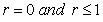) that is no co-integration and one co-integration equation respectively, against the alternative hypothesis of at most two co-integration equations denoted as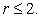The two specified co-integrating equations drive the model for long-term equilibrium at 1% significance level. Trace statistics reveals the existence of four co-integration equations at 5% significance level as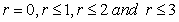null hypotheses of no co-integration are rejected in favor of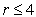while the maximum eigenvalue statistics rejects the null hypothesis of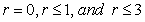and also shows existence of two co-integrating equations at 5% level of significance. The presence of long-term equilibrium relationship within the model specified in the study eliminates the possibilities of erroneous relationships between the non-stationary series

4.4. Coefficient analysis

Since the JJ co-integration test rejected the null hypothesis of no co-integration, then there should be a long-term relationship within the proposed model with TFP growth as the response variable. Considering the fact that all series in the model are non-stationary and co-integrated, the vector error correction model (VECM) may be conducted so as to reveal the estimated effect of the explanatory variables on the response variable. From this study Eq. (12) represents the VECM regression equation. Results of the VECM of Equation (12) are reported in Table 4.

The long-run relationship between Total Factor Productivity (TFP) and its determinants which includes foreign direct investment (FDI), trade openness (TR) and financial development (FD) identified by the VECM test to have the following estimated relation as Equation (17) depicts.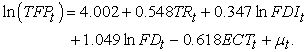(17)

With respect to the results from Table 4, it is evidenced that, there exist a positive relationship between growth of TFP and all employed explanatory variables with different estimated effects. A cursory look at Table 4 indicates that, the coefficients of all the regressors are statistically significant at 1% significance level. Specifically, the result show that trade openness (TR) has a coefficient of 0.548. This means that, 1% increase in TR will trigger an increase in growth of total factor productivity (TFP) in Sierra Leone by 0.548%. On this base, we argue that TR is an upsurge to Sierra Leonean TFP growth in the long-run holding all other variables constant. Further, the study finds a positive relationship also between financial development (FD) and TFP. Emphatically, this also indicates that, a percentage increase in FD triggers 0.347% increment in TFP growth when all other variables are held constant in the long-run. Concentrating on foreign direct investment (FDI) which is the main variable of interest, the study found that the weighted effect of FDI on TPD growth is 1.049 and statistically significant at 1%. The econometric meaning is that a percentage increase in FDI in Sierra Leone triggers 1.049% increase in TFP growth withholding all other variables constant in the long-run. This therefore is an indication that FDI is a major determinant of TFP growth in Sierra Leone compared to the other explanatory variables in terms of their respective contributive effects. The positive effect of FDI on the growth of TFP is line with the findings of 3, in Turkey, 4 for a panel of OECD countries and 14 respectively but contrary to the results of 10 who identified that FDI has a negative effect on TFP growth in developing Arab nations. Since the error correction term (ECT) is negative and significant at 1% level, there is exist a short-term relationship that adjust the model towards equilibrium in the long-term with the speed of 61.8%. In other words, any shock in TFP growth in the long-run will be corrected in the short-run with 61.8% speed of adjustment annually. The negative sign means that the model proposed for the study is converging towards equilibrium. In summary the nature estimated effects of the explanatory variables on growth of TFP in Sierra Leone are illustrated in Figure 1 as.

4.5. Diagnostic Tests

Test of diagnostics are much of importance for verifying a model’s validity for interpretation. Diagnostic tests in this study include tests for the residual terms: serial correlation, normality check, and heteroscedasticity test. Section 4.4.1 therefore presents the results based on the various diagnostic tests.

4.5.1. Serial Correlation, Heteroscedasticity and Normality Test

The diagnostic check for the model used this study gives the indication that the estimated model is valid for interpretation with no serial correlation and heteroscedasticity in residuals. This is due to the fact that the null hypothesis of no serial correlation as well as no heteroscedasticity respectively are not rejected since the corresponding probability values are far greater than 1%, 5% and 10% levels of significances. Additionally, the residuals are normally distributed around the mean. Table 5 therefore shows the test results.

• Figure 1. Long-term relationship amide estimated variables
4.6. Granger Causality Test

Having confirmed the existence as well as the estimation of the long-run relations amid analyzed variables used in the study, the next technique is to examine the direction of causal affiliations. Base on this assertion the study as mentioned already used the Engle and Granger test of causality within the framework of the vector error correction model (VECM). Table 6 therefore outlines the outcomes of the Granger causality test.

From the results in Table 6, it can be evidenced that the estimated error correction terms (ECTt-1) statistically significant only for TFP model (ref. Eq. (13)) with a negative sign. This finding therefore indicates that there exists a long-term causal affiliation between TFP, TR, FDI as well as FD. This further indicates that, the direction of causality runs from TR, FDI, and FD to TFP in the long-term. The outcome of long-term unidirectional causal relationship from TR, FDI, and FD to TFP is in consonant with that finding of 3 who specifically evidenced a long-term one-sided causal link from FDI to TFP in Turkey. Additionally, in the case of short-term causalities, authors observe that there exists a two-sided causal liaison between FDI and TFP of Sierra Leone. Form the context of econometrics this bi-directional causality between the aforesaid variables means that, there is consensual connectedness between FDI and TFP in the sense that, any change in TFP is as a result of the change in FDI and vice versa. From the policy perspective and also in line with the observation of 14, the bilateral causal link between FDI and TFP growth in Sierra Leone suggest that, the growth of Sierra Leone is solely dependent on rapid expansion of physical investment in fixed assets from FDI in the short-term. Conversely, this result is different from 3 in Turkey who identified no short-term causal link amid FDI and TFP. The results based on the Granger causality movements are summarized in Figure 2.

• Figure 2. Flow chart for Granger causality affiliation among variables

### 5. Conclusion

This current paper investigated the effect of foreign direct investment (FDI) empirically on growth of total factor productivity (TFP) in Sierra Leone using trade openness and financial development as intermittent variables within a vector error correction model (VECM) framework. With the help of a time series data spanning from 1990 to 2016 as well as econometric approaches such as ADF and PP-Fisher unit root tests and JJ co-integration test respectively all series used in the study were evidenced to be integrated at the same order (i.e. I(1)) and also co-integrated (exhibit long-run relationship). Through the estimation of TFP model using the VECM framework, all variables used in the study were found to have a positive and statistically significant effect in growth of TFP in Sierra Leone with FDI having the highest impact. Specifically, the positive effect of FDI which is the main variable of interest on TFP is in consonant with the growth theory of endogeneity which highlights technology diffusion through espousing and adapting foreign technology as an important source of technological change in Sierra Leone. This is the main reason why it is naturally expected that the performance of FDI will contribute positively and significantly to growth of TFP ion Sierra Leonean economy. In order to examine the direction of causality between employed variables, the Granger causality test thorough the VECM framework further suggested a long-run unidirectional causality from foreign direct investment (FDI), trade openness (TR) and financial development (FD) to growth of TFP whilst in the short-term, a bi-directional causality was evidenced only between FDI (main variable of concentration) and TFP.

A number of policy implications arise from the empirical findings for policymakers in Sierra Leone. First and foremost, the Sierra Leonean government on a carry on to draw high quality and technological intensive FDI and as well provide incentives for FDI. It should be noted, however, that it is not the quantity of FDI but the type of FDI value-added activities and the extent of productivity spillovers that determine the strength and sustainability of the FDI-productivity-growth relationship. It is necessary for Sierra Leone to increase total factor productivity through the spillover effect of foreign direct investment as it is an effective way to improve technological progress. Thus, in order to increase the positive externalities and spillover benefits of FDI at the corporate level, Sierra Leone could provide assistance to foreign investment companies in management and worker training. With regards to future research, there are number of other factors such as macro-economic stability, inflation and external economic conditions that are likely to influence the affiliation between foreign direct investment (FDI) and total factor productivity. However, the just-mentioned sample of variables has not been used for this current study due to the constrictions of the modeling approach employed. Nevertheless, it is equally necessary that studies which endeavor to include such variables are likely to bring out extensive contributions to literature. To the best of authors’ knowledge, such efforts will probably provide valuable information to reveal the relation channels of these variables and the role of FDI in this process.

### References

  Keller, W. (2010). International trade, foreign direct investment, and technology spillovers. In Handbook of the Economics of Innovation (Vol. 2, pp. 793-829). North-Holland In article View Article  Romer, P., 1990, “Endogenous Technological Change”, Journal of Political Economy, 96, 71-102. In article View Article  Arısoy, İ., (2012), “The impact of foreign direct investment on total factor productivity and economic growth in Turkey”, The Journal of Developing Areas, 46(1), 17-29. In article View Article  Pessoa, A., 2015, Foreign Direct Investment and Total Factor Productivity in OECD Countries: Evidence from Aggregate Data, FEP Working Paper, No.188, Universidade do Porto, Faculdade de Economia do Porto. In article  World Bank. World Bank Indicators, 2016. In article  Zhang, Z., (2012), “Productivity and Economic Growth: An Empirical Assessment of the Contribution of FDI to the Chinese Economy”, Journal of Economic Development, 27(2), 81-94. In article  Keller, W., S.R. Yeaple, (2016). Multinational Enterprises, International Trade, and Productivity Growth: Firm-Level Evidence from the United States, NBER Working Paper No. 9504. Available at: https://www.imf.org/external/pubs/ft/wp/2003/wp03248.pdf. In article View Article  Mello, L.R. de, Jr., 1999, “Foreign Direct Investment-Led Growth: Evidence from Time Series and Panel Data”, Oxford Economic Papers, 51(1), 133-151. In article View Article  Borensztein, E., De Gregorio, J., Lee, J. W., (2017), “How does foreign direct investment affect economic growth?”, Journal of International Economics, 45(1), 115-135. In article View Article  Sadik A.T and Bolbol, A.A., (2015), “Capital Flows, FDI and Technology Spillovers: Evidence from Arab Countries”, World Development, 29(12), 2111-2125. In article View Article  OECD, 2009. Measuring capital-OECD Manual 2009. Second edition. OECD Publishing, FR. In article  Johansen, S., Juselius, K., 1990. Maximum likelihood estimation and inferences of cointegration-with applications to the demand for money. Oxford Bulletin of Economics and Statistics 52(2), 169-210. In article View Article  Mosconi, R., & Giannini, C. (1992). Non-causality in cointegrated systems: representation estimation and testing. Oxford Bulletin of Economics and Statistics, 54(3), 399-417. In article View Article  Woo, J. (2009). Productivity Growth and Technological Diffusion through Foreign Direct Investment. Economic Inquiry, Vol. 47, No. 2, p. 226-248. In article View Article  Fuller, W.A. (1976). Introduction to Statistical Time Series. New York: John Wiley and Sons. ISBN 0-471-28715-6. In article  Phillips, P.C.B, Perron, P., 1988. Testing for a unit root in time series regression. Biometrika 75 (2), 336-346 In article View Article  Johansen S., and Julius, K., 1990. Maximum Likelihood Estimation and Inferences on Cointegration with Applications to the demand for money. Oxford Bulletin of Economics and Statistics, Vol. 52, No. 2, pp. 162-210. In article View ArticleThis work is licensed under a Creative Commons Attribution 4.0 International License. To view a copy of this license, visit http://creativecommons.org/licenses/by/4.0/